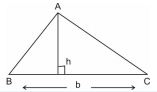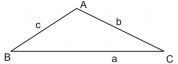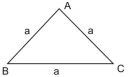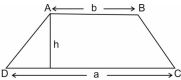### Herons Formula - Revision Notes

CBSE Class 09 Mathematics

Revison Notes
CHAPTER 12
HERON’S FORMULA

1. Area of a Triangle – by Heron’s Formula

2. Application of Heron’s Formula in finding Areas of Quadrilaterals

• Triangle with base 'b' and altitude 'h' is• Area of an isosceles triangle whose equal side is $a$ = $\frac{{a}^{2}}{2}$ square units
• Triangle with sides a, b and c

(i) Semi perimeter of triangle s =$\frac{a+b+c}{2}$

(ii) Area=$\sqrt{s\left(s-a\right)\left(s-b\right)\left(s-c\right)}$sq. unit• Equilateral triangle with side 'a'

Perimeter = $3a$ units

Altitude = $\frac{\sqrt{3}}{2}a$ units• Rectangle with length $l$, breadth $b$

Perimeter = $2\left(l+b\right)$

Area = $l×b$

• Square with side $a$

Perimeter = $4a$ units

Area = ${a}^{2}$  sq. units

Area = ${\left(\mathrm{D}\mathrm{i}\mathrm{a}\mathrm{g}\mathrm{o}\mathrm{n}\mathrm{a}\mathrm{l}\right)}^{2}$ sq. units

• Parallelogra with length $l$, breadth $b$ and height $h$

Perimeter = $2\left(l+b\right)$

Area = $b×h$

• Trapezium with parallel sides 'a' & 'b' and the distance between two parallel sides as 'h'.